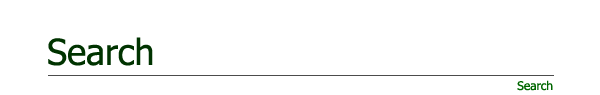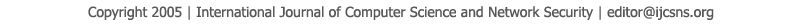To search, Click below search items.All Published Papers Search Service
 Title Author Year Keyword
 Title Comparison Logistic Regression and Discriminant Analysis in classification groups for Breast Cancer Author Krieng Kitbumrungrat Citation Vol. 12  No. 5  pp. 111-115 Abstract This research is a model group, the probability that a patient is detected at any breast cancer or not breast cancer. Assessment of characteristics of abnormal growth of breast cancer cells such as Clump Thickness (X1), Uniformity of Cell Size (X2), Uniformity of Cell Shape (X3), Marginal Adhesion (X4), Single Epithelial Cell Size (X5), Bare Nuclei (X6), Bland Chromatin (X7), Normal Nucleoli (X8), and Mitoses (X9) are independent variable. The dependent variable is the probability that the patient is detected at any breast cancer or not breast cancer by using Logistic Regression Model and Discriminant Model. Conclude that Logistic Regression Model has 96.90% classification higher than Discriminant Model has 96.10% classification. Logistic Regression Model can used predicted variables 4 variables are Clump Thickness (X1), Marginal Adhesion (X4), Bare Nuclei (X6) and Bland Chromatin (X7). So the model in predicting the probability of collection for the classification of patients breast cancer is ln = 10.104 - 0.535X1 - 0.331X4 - 0.383X6 -0.447X7. The study results reveal that the discriminant analysis can used predicted variables 9 variables for classifying groups of breast cancer and non- breast cancer. So the factor model for the classification of Fisher's linear discriminant functions affecting the breast cancer model. The classifying groups of breast cancer is D1=-23.536+1.63X1+0.312X2+0.495X3+0.339X4+0.995X5+ 1.457X6+1.198X7+0.547X8 and the classifying groups of not breast cancer isD2=-3.288+0.742X1+0.301X2+0.057X3+ 0.106X4 +0.708X5 +0.188X6 +0.664X7 +0.026X8. Keywords Breast cancer, Logistic Regression Model, Discriminant Model URL http://paper.ijcsns.org/07_book/201205/20120518.pdf